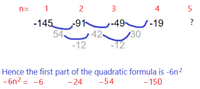# How is -1 the next number in this sequence -145, -91, -49, -19?

#### Kessen

##### New member
How is -1 the next number in this sequence -145, -91, -49, -19?
What is the full formula of the quadratic sequence and how is it derived?
So far I have deciphered the first part of the quadratic formula as -6n2 by using the details shown below.
View attachment 15929
Thank you
-

#### Subhotosh Khan

##### Super Moderator
Staff member
How is -1 the next number in this sequence -145, -91, -49, -19?
What is the full formula of the quadratic sequence and how is it derived?
So far I have deciphered the first part of the quadratic formula as -6n2 by using the details shown below.
View attachment 15929
Thank you
Nothing shown above!!

#### MarkFL

##### Super Moderator
Staff member
How is -1 the next number in this sequence -145, -91, -49, -19?
What is the full formula of the quadratic sequence and how is it derived?
So far I have deciphered the first part of the quadratic formula as -6n2 by using the details shown below.
View attachment 15929
Thank you
-
The first differences are:

-54, -42, -30

And the second difference is constant at -12. So, the next first difference will be -18, which makes the next term -1.

Since the second difference is constant, we will indeed have a quadratic for the $$n$$th term. So, we may use the given values to obtain a system of equations:

$$\displaystyle a_1=a+b+c=-145$$

$$\displaystyle a_2=4a+2b+c=-91$$

$$\displaystyle a_3=9a+3b+c=-49$$

Can you proceed?

#### Kessen

##### New member
I cannot proceed. The first part of the quadratic is -6n2 but the latter terms remain unknkown.

For the 5th term in the original sequence, (term a5) where n=5 and we know that a5 = -1
a5= −6n2+ ?n + ? = −1The difference between the expected and acheived terms is as follows

 My sequence as detailed above in red (-6n2) -6 -24 -54 -150 Original sequence -145 -91 -49 -19 The difference between my sequence and the original sequence is inconsistant as shown here > -151 -115 -103 -169

Last edited:

#### MarkFL

##### Super Moderator
Staff member
Using a CAS, I get:

$$\displaystyle (a,b,c)=(-6,72,-211)$$

And so:

$$\displaystyle a_n=-6n^2+72n-211$$

#### Dr.Peterson

##### Elite Member
I cannot proceed. The first part of the quadratic is -6n2 but the latter terms remain unknkown.

For the 5th term in the original sequence, (term a5) where n=5 and we know that a5 = -1
a5= −6n2+ ?n + ? = −1

View attachment 15935
The difference between the expected and acheived terms is as follows

 My sequence as detailed above in red (-6n2) -6 -24 -54 -150 Original sequence -145 -91 -49 -19 The difference between my sequence and the original sequence is inconsistant as shown here > -151 -115 -103 -169

Your fourth term is wrong; -6(4)^2 = -96, not -150. Also, you added rather than take the differences.

But your method is not the only way to solve the problem. Did you not understand post #3, which gave you a system of equations to solve for the coefficients?

#### HallsofIvy

##### Elite Member
First there are an infinite number of perfectly valid continuations of that sequence. To find one you can use the fact that, given n points, there exist a unique polynomial, of degree n-1 or less, passing through those n points.

Here, taking the "x" values to be 0, 1, 2, and 3, we have four points (0, -145), (1, -91), (2, -49), and (3, -19) so we can find a polynomial of degree 3, $$\displaystyle y= ax^3+ bx^2+ cx+ d$$. Taking x= 0, y= -145, $$\displaystyle a(0^3)+ b(0^2)+ c(0)+ d= d= -145$$. Taking x= 1, y= -91, $$\displaystyle a(1^3)+ b(1^2)+ c(1)+ d= a+ b+ c+ d= -91$$, $$\displaystyle a(2^3)+ b(2^2)+ c(2)+ 2= 8a+ 4b+ 2c+ d= -49$$, and $$\displaystyle a(3^3)+ b(3^2)+ c(3)+ d= 27a+ 9b+ 3c+ d= -19$$.

Since d= -145, we have a+ b+ c- 145= -91 so a+ b+ c= 54, 8a+ 4b+ 2c- 145= -49 so 8a+ 4b+ 2c= 96, and 27a+ 9b+ 3c- 145= -19 so 27a+ 9b+ 3c= 126.

Now we have three linear equations to solve for a, b, and c.